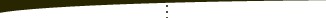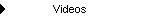About   Products   Support   Videos   Purchase   ContactThe Breakout Bulletin

The following article was originally published in the December 2006 issue of The Breakout Bulletin.

### Trading Rules from Statistical Grouping of Indicators

A while back I started thinking about the mathematical characteristics of neural networks. One of their key characteristics is that they use a complex, nonlinear function to capture patterns in the market that are not obvious with more conventional methods. The nonlinear function combines a set of inputs, typically based on indicators (e.g., moving averages, stochastics, momentum, and so on), into a single output that ranges from -1 to +1. To use this output in trading, we can look for long entries when the function output is above +0.5 and for short entries when the function output is below -0.5.

Transforming the multiple inputs into a single output is a continuous, nonlinear function. Trying to fit this single continuous function to different sets of market conditions simultaneously so that the network properly assigns bullish conditions to output values greater than 0.5 and bearish conditions to output values less than -0.5 is quite challenging, to say the least. In my experience there are many different sets of conditions that set up profitable long trades and many other sets of conditions that set up profitable short trades. Perhaps it's asking too much of a single continuous function to somehow weave a twisted path among disparate sets of market conditions.

This led me to wonder if the neural network approach could be adapted to "discrete"-value functions. In other words, rather than using a continuous function, the function would have a small number of values, perhaps each one corresponding to a different set of market conditions. One way to approach this is by grouping the data into "bins," similar to the method I used in the article on Dynamic Portfolio Selection.

As an example, suppose we have the following set of five indicators:

I1 = C - C

I2 = C - C

I3 = C - AMA

I4 = AMA - Average(C, 30)

I5 = TrueRange - Average(TrueRange, 30)

where C is the closing price of the current bar, C is the close one bar ago, C is the close three bars ago, AMA is an adaptive moving average based on a variable speed exponential moving average, as calculated by the TradeStation built-in function AdaptiveMovAvg(C, 20, 2, 40), Average(C, 30) is the simple moving average of the last 30 closing prices, TrueRange is the true range of the current bar, and Average(TrueRange, 30) is the simple moving average of the true range over the last 30 bars.

If we determine the minimum and maximum values for these indicators over our data set, we can scale the indicators so that they lie in the range -1 to +1, denoted [-1, +1]. For example, to scale I1 into this range, we can write:

I1scale = (2 * I1 - (SfMax1 + SfMin1))/(SfMax1 - SfMin1)

where SfMax1 and SfMin1 are the maximum and minimum values, respectively, of I1.

Now that the indicators all produce values in the range [-1, +1], we can group them into bins based on their values. Suppose we divide the range into the following four bins: [-1, -0.5], (-0.5, 0], (0, +0.5], (+0.5, 1]. So, if an indicator is greater than or equal to -1 and less than or equal to -0.5, it lies in bin #1, [-1, -0.5]. If the indicator is greater than -0.5 and less than or equal to zero, it lies in bin #2, (-0.5, 0]. Values greater than zero and less than or equal to 0.5 lie in bin #3, (0, +0.5]. Finally, if an indicator is greater than 0.5 and less than or equal to 1, it lies in bin #4, (+0.5, 1].

The basic idea of this approach is to evaluate the five indicators on the bar prior to each trade we want to take. To keep things simple for illustrative purposes, I'll assume the trades enter on the day's open and exit at the close on the same day. Provided we're using intraday data, this means we want to evaluate the indicators on the last bar of each day in preparation for entering on the next day's open.

When we evaluate the indicators, we'll determine into which bin each one falls. We'll keep track of the bins for each indicator for each trade. We'll also keep track of the profit/loss amount for each trade. At the end, we'll find the combination of bins that produced the greatest net profit. For example, it might turn out that when the five indicators were in bins 3, 1, 2, 1, and 4, respectively, we obtained the most profit buying on the open (i.e., going long). By taking the negative value of the profit/loss amounts, we can use the same data to find the best bin combination for going short on the open.

Notice that the number of possible bin combinations is the number of bins raised to the power of the number of indicators, or 4^5 = 1024 combinations in our example. It will turn out that only a relatively small number of these combinations contain more than one trade. We want to focus on the combinations that contain a reasonably large number of trades so the results will be more significant.

I wrote a strategy in TradeStation called StatBins1 to perform the calculations. This strategy can be downloaded from my web site on the free downloads page. Instructions for applying the strategy are provided in the lengthy comment at the top of the strategy code.

After finding the scaling factors, I ran StatBins1 on 60 minute bars of the e-mini Russell 2000 futures over the past five years, ending 12/15/2006, with a lookback length of 50. The strategy produced the following results, which were written to the TradeStation Print Log:

StatBins1...
Total Number of Simulated Trades = 1239
Combo      Total Long P/L       No. Trades
22         4550.00          7
23         -1390.00          3
27         -510.00          4
66         -1100.00          2
70         1170.00          32
71         -2570.00          5
82         530.00          2
86         2810.00          97
87         -5860.00          41
90         -14130.00          46
91         480.00          82
102         -5160.00          28
103         1520.00          14
106         -8890.00          60
107         -2650.00          133
111         -3830.00          10
123         -840.00          8
127         -580.00          3
134         -690.00          21
135         3800.00          6
139         2170.00          2
146         -2100.00          2
150         6420.00          76
151         6210.00          34
154         2280.00          38
155         8000.00          46
166         3220.00          26
167         3000.00          15
170         -4710.00          81
171         -10100.00          158
186         300.00          2
187         990.00          14
234         1810.00          10
235         1330.00          14
326         -630.00          10
342         -1920.00          14
343         660.00          3
346         160.00          3
347         680.00          6
363         1320.00          10
367         -830.00          2
386         220.00          3
390         1370.00          5
406         -1060.00          9
410         1140.00          2
411         1470.00          7
422         1090.00          2
426         2120.00          3
427         -920.00          14
491         1520.00          3

Bins (0 - 3): [-1, -.5] (-.5, 0] (0, .5] (.5, 1]
Maximum Profit/Loss, Long: 8000.00
Bins:   2  2  1  2  0

Maximum Profit/Loss, Short: 14130.00
Bins:   1  2  1  1  0

The first part of the output is a table of bin combinations, with the combinations numbered from 1 to 1024.The table lists the net profit and number of trades for bin combinations with more than one trade. Combinations with positive net profit amounts represent net profitable long trades, whereas negative amounts represent net profitable short trades. Following the table, the combination with the greatest net profit is shown for both long and short trades. For example, the best bin combination for long trades was when indicator I1 was in bin 2, indicator I2 was in bin 2, indicator I3 was in bin 1, indicator I4 was in bin 2, and indicator I5 was in bin 0 (the bins are numbered from 0 to 3 in the strategy).

To see more detailed performance results for the optimal bin combinations, theStatBins1 strategy can optionally be run as a system by entering the optimal bin numbers as inputs to the strategy and setting the input "TradeIt" to TRUE. When this is done, StatBins1 uses the indicators and bin numbers to take trades when the indicators lie in the specified bins. Trades are entered at the open and exited at the close. No stop is used.

With \$25 per round turn deducted for slippage and commissions, StatBins1 produced the following results on a one-contract basis using the optimal bin combinations shown above:

Net Profit: \$19,830

Profit Factor: 2.28

Percent Profitable: 63.0%

Ave Win/Ave Loss: 1.34

The equity curve is shown below in Fig. 1.Figure 1. StatBins1 optimal trading results on ER2, 60 min, five years ending 12/15/06, with \$25 trading costs.

Although in the example presented here, the indicator bins were used as the sole trade entry criterion, it's probably better to combine this approach with other entry and exit techniques. For example, the StatBins1 code could be modified to track the bins prior to entering trades on a breakout or using other criteria. In that way, the indicator bin values would serve as a filter for the primary entry technique. More sophisticated exit techniques could be added as well.

Also note that the results shown above are in-sample, optimized results. Using the bin values that generate the greatest net profit is optimization. Out-of-sample testing and real-time tracking are always a good idea before committing real money to any new trading approach.Copyright © 2001-2013 Breakout Futures Copyright © 2013-2017 Adaptrade Software